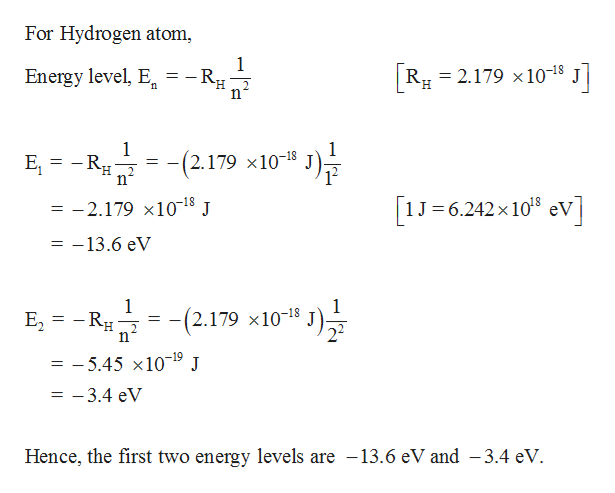As the interaction between the nucleus and the electron determines the structure of the energy levels of the H atom, any quantum object that has a positively and a negatively charged particle with no other interactions should have a similar structure of energy levels. Consider a very unusual 'H atom', where the positevely charged particle is a positron (the antimatter particle of the electron, same mass as the electron, but opposite charge). Calculate the first two energy levels for this unusual atom in eV. What wavelength (in nm) for a transition between these two energy levels?

Question

As the interaction between the nucleus and the electron determines the structure of the energy levels of the H atom, any quantum object that has a positively and a negatively charged particle with no other interactions should have a similar structure of energy levels. Consider a very unusual 'H atom', where the positevely charged particle is a positron (the antimatter particle of the electron, same mass as the electron, but opposite charge). Calculate the first two energy levels for this unusual atom in eV. What wavelength (in nm) for a transition between these two energy levels?

Step 1

The first two energy levels are...help_outlineImage TranscriptioncloseFor Hydrogen atom 1 - Rg R 2.179 x 1018 J Energy level, E n 1 - Rg -2.179 x10-18 _ H 2 n 1 J 6.242x 108 eV -2.179 x1018 J 11 X -13.6 eV 11 1 E2 RH -Ru고- -(2.179 x10-18 = -5.45 x10-19 J - -3.4 eV 11 Hence, the first two energy levels are -13.6 eV and -3.4 eV fullscreen

Want to see the full answer?

See Solution

Want to see this answer and more?

Our solutions are written by experts, many with advanced degrees, and available 24/7

See Solution
Tagged in

Physical Chemistry